ISSN ONLINE(2320-9801) PRINT (2320-9798)

All submissions of the EM system will be redirected to Online Manuscript Submission System. Authors are requested to submit articles directly to Online Manuscript Submission System of respective journal.

# Energy Histogram for Image Retrieval using Relevance Feedback

 Subashini.M, Narayanan.R.C, Dr.K.Krishnamoorthy PG Scholar, Department of CSE, Sona College of Technology, Salem, Tamil Nadu, India Associate Professor, Department of CSE, Sona College of Technology, Salem, Tamil Nadu, India HOD, Department of CSE, Sundharsan Engineering College, Pudukkottai, Tamil Nadu, India Related article at Pubmed, Scholar Google

Visit for more related articles at International Journal of Innovative Research in Computer and Communication Engineering

## Abstract

This paper presents the novel approach by combining effective techniques in Content-Based Image Retrieval technique. By considering CBIR system, effective retrieval is achieved by using relevance feedback mechanism. The issue in effective retrieval needs an effective extraction of low-level features, which encompasses on image such as color, texture and shape. These pave way for fast and easy indexing of query image with the database image. In current trend most of the images are compressed and stored in the form of JPEG using Discrete Cosine Transformation (DCT). The proposed method is being designed in such a way to produce most precise result by taking user feedback into account with different histogram quantization in a compressed domain.

### Keywords

Content-based image retrieval (CBIR) ,Quantized histogram, SVM Classifier, Relevance Feedback.

### INTRODUCTION

Human perception is more likely towards images rather than text. Currently in the field of modern communication system, visual communication plays an important role. In internet the vast storage space has to be effectively managed by storing the images in a compressed form. For handling the vast amount of image collection, the necessity for effective retrieval came into account. Therefore effective image retrieval is still an open challenge. In general there are three basic techniques for image retrieval they are text-based, content-based and semantic-based. In text-based image retrieval, the retrieval process requires the textual description and annotation that makes the retrieval process tedious and time-consuming.
CBIR  plays an active role in the field of research for producing effective image retrieval. They are effectively boosted by combining it with the relevance feedback mechanism. The CBIR is performed in two stages. The basic stage is known as indexing in which the features of images are being extracted and they are stored in the feature database. Initially the images are decoded from compressed  domain to pixel domain to extract the low level features. The next stage is known as searching in which user query images feature vector is compared to retrieve the similar images available in the feature database. Since the extraction of features is tedious in compressed domain we go for Discrete Cosine Transformation  which is a part of image compression. In the compressed process the DCT removes little information from the image and allow the accessible information behind which helps in effective image retrieval.
This leads to CBIR  in which textual description is avoided and low-level features has been analyzed for making faster computation. The semantic gap between low-level features  and high-level concepts handled by the user in image retrieval needs to achieve reasonable solutions for effective retrieval especially in compressed domain. This is achieved by inculcating various distance metrics which are used to measure similarities using texture features and also various distance metrics is performed in every individual quantization bins.
In the initial stage the image is being converted from RGB to YUV color model and then they are divided into 8x8 DCT blocks using DC and AC coefficients. These blocks are then used to extract the texture feature using YUV color model in which the images is split into four blocks and they Y component in each block is transformed into DCT coefficients to get vertical, horizontal and diagonal texture features in all blocks for effective representation. The texture features from the image are being extracted in compressed domain by computing mean and standard deviation using DCT coefficient. This result is used to form the feature vector to retrieve similar images.In this paper the major concern is about utilizing the quantized histogram statistical texture feature effectively by matching the query image with the database image using various distance metrics of the DCT domain. After the effective feature extraction they are being quantized into different number of bins. The performance is analyzed and the resultant image is being displayed.
The Manhattan Distance (L1 metric) gives the high performance in terms of precision among various technique such as the Euclidean Distance (L2 metric) and the Vector Cosine Angle Distance (VCAD) to measure the distance for similarity between query image and the images available in the image database. In this method the comparison methods are done in two types. First the distance metrics measures the difference between the two vectors of images and the similarity is determined by the small difference between them. In the similarity measure metrics, the similarity between the two vectors of images and a large similarity means the two images are closely similar to each other.
In the proposed work this retrieved similar images is given to the SVM classifier  which classifies the output into relevant and irrelevant images. Finally the user feedback is taken into account by considering the retrieved relevant images from the SVM classifier to obtain the most precise output. This proposed technique is being elaborated in the following sections.

### BACKGROUND METHODOLOGY

A. Feature Extraction
Feature extraction  is the basis of content based image retrieval. Typically two types of visual feature in CBIR:
1) Primitive features which include color, texture and shape.
2) Domain specific which are application specific and may include, for example human faces and finger prints.
B. Histogram quantization
The histogram of the DC is defined as the frequencies of the DC coefficients and the histogram of the AC is the frequencies of the AC coefficients in all the blocks. The DC histogrameq(1) is then quantized into L bins such that: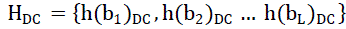where h(bi)DC is the frequency of the DC coefficients in bin biand HDC is the histogram of the L bins. The histograms for the AC coefficients are eq(2-4) also quantized into L bins such that: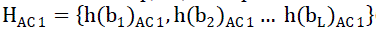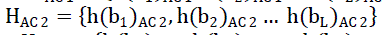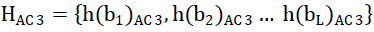whereh(bi)AC 1, h(bi)AC 2 and h(bi)AC 3 are the frequencies and HAC 1, HAc 2 and HAC 3are the histograms of AC1, AC2 and AC3 coefficients using the quantization of the L bins.

### PROPOSED SYSTEM

A. Image Segmentation
The initial stage of my system is to convert RGB input images to YUV color model. This color model helps in effective retrieval of images in a compressed domain . After the conversion of the color model they are being compressed in order to store it effectively. During the compression DCT technique is being used in order to segment the given image into 8x8 block transformation
B. Feature Extraction
The next stage after segmentation is that, considering 2x2 blocks to identify the histogram for effective retrieval. Each block is considered as four co-efficient namely DC, AC1, AC2 and AC3. DC components are considered as the energy component and other AC components are taken into consideration while constructing the feature vector. Then the identified histograms are quantized into 8, 16 and 32 histogram quantization bins. Finally the texture feature is computed in each histogram and the feature vector  is constructed. The stastical texture features like mean and standard deviations are computed.
Let P(b) be the probability distribution eq(5) of bin b in each of the histograms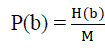where M is total number of blocks
The mean eq(6) is the average of intensity values of all bins of the four quantized histograms. It describes the brightness of the image (Szabolcs, 2008; Selvarajah and Kodituwakku, 2011) and can be calculated asThe standard deviation eq (7) measures the distribution of intensity values about the mean in all blocks of histograms. The calculated value of standard deviation shows low or high contrast of histograms in images with low or high values (Selvarajah and Kodituwakku., 2011; Thawari and Janwe, 2011) and is calculated as: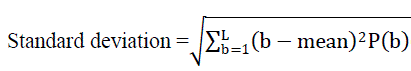C. Similarity Measure
In this phase the CBIR system identifies the similarities between images by using distance metrics. This distance metricseq(8-11) which are considered in my work are the sum of absolute difference (SAD), the sum of squared of absolute differences (SSAD), Euclidean distance and city block distance (Manhattan distance). They measure the similarity between the query image and the image available in the database. The small difference between the two feature vector indicates the large similarity and vice versa.
1) Sum of Absolute Difference (SAD): The sum of absolute difference (SAD)3eq(8) is a very straightforward distance metric and extensively used for computing the distance between the images in CBIR to get the similarity. In this metric the sum of the differences of the absolute values of the two feature vectors are calculated (Selvarajah and Kodituwakku, 2011). The similarity is decided on the computed value of distance. This distance metric can be calculated as: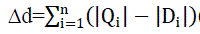Where n is the number of features, i= 1, 2. . ., n. Both images are the same for Δd = 0 and the small value of Δd shows the relevant image to the query image.
2) Sum of Squared Absolute Difference (SSAD): In this metric the sum of the squared differences of absolute valueseq(9) of the two feature vectors are calculated. This distance metric can be calculated (Selvarajah and Kodiyuwakku, 2011) as: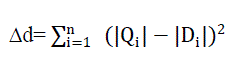3) Euclidean distance:This distance metric eq(10) is most commonly used for similarity measurement in image retrieval because of its efficiency and effectiveness. It measures the distance between two vectors of images by calculating the square root of the sum of the squared absolute differences and it can be calculated (Szabolcs, 2008) as: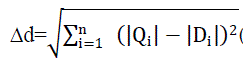*) (10)
4) City block distance:This distance metriceq(11) is also called the Manhattan distance. The city block distance metric has robustness to outliers. This distance metric is computed by the sum of absolute differences between two feature vectors of images and can be calculated (Szabolcs, 2008) as: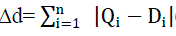(11)
D. SVM Classifier
Support Vector Machines  are a set of related supervised learning method used for classification and regression SVM predicts that the new image falls into one category or the other. In out method this classifier is used to classify the images into relevant and non-relevant images from the set of retrieved output images.
E. Relevance Feedback
The technique used for relevance feedback is the random walks in which they depend upon the random walker algorithm . This technique uses the classified relevant images as the input and gets user feedback into account for generating the most precious output. They also have some iteration until the user gets their required output.
F. Proposed Algorithm
Step 1: Initially, user query images and images from the image collection is seeded as the input.
Step 2: In the next level, the RGB color images are converted to YUV color model
Step 3: Then the DCT block transformation is being carried out as one of the image compression technique.
Step 4: Then histogram identification of DC, AC1, AC2, AC3 co-efficient is applied in all the blocks
Step 5: The identified histogram are quantized into 8, 16 and 32 histogram quantized bins.
Step 6: Texture feature is computed in each histogram and the feature vector is constructed.
Step 7: CBIR system identifies the similarities (similarity measure) between the images using distance metrics.
Step 8: System retrieves the set of images for the given query image on which SVM classifier is used to classify the images into relevant and non-relevant images
Step 9: On the set of retrieved relevant images, user is going to give feedback to find the most precious output and return back to step 6.
Step 10: If the feedback is satisfactory, then system retrieves user required image set. If not the feedback process is iterated.

### EXPERIMENTAL RESULT

The coral dataset is being used to test the improvised performance of the proposed system, which is available for researchers. The image database consists of 14,500 images having 55 categories each of which has approximately 260 images. The sample categories are Chihuahua, Pekinese, Shih-Tzu, Papillion, Basset, Beagle, Bluetick and Redbone. All the above mentioned and 55 specified categories are used for the experiment. All the images are initially stored in RGB color space in compressed format. They are approximately compressed to 2KB effectively using DCT transformation which helps in retaining of useful information for effective extraction.
A. Metrics Measurements
The rapid change in development of the technology people are now more attracted to images rather than text documents therefore currently storage of huge amount of images in the databases is a big challenge. This is being overcome by the one of the effective image compression technique known as Discrete Cosine Transformation(DCT) in which even after compression the useful information are being retained. This paves ways for effective extraction of features from the DCT block of images. The similarity between the query image feature vector and the feature vector available on the feature database are being compared using precision metrics which is considered to be one of the important techniques.
1) Precision:The precision in image retrieval determines the amount of relevant images retrieved from the total number of retrieved images for the given query image.
Precision = Relevant image retrieved / Retrieved image
Table 1 shows the average precision of sample image categories in different quantized histogram bins using city block (Manhattan) distance which is considered to be the most precious metrics in terms of precision. It explains by the value that there is an improvement when the number of bins increases and which reaches the maximum when the bin value reaches 32 which show an average of 91% and the overall percentage achieved is 90%, which records the better performance. Fig 2 shows the graphical representation of the value in the table present

### CONCLUSION

The CBIR method which is proposed is based on the performance analysis of various distance metrics using the quantized histogram statistical texture features in the DCT domain. The similarity measurement is performed by using four distance metrics. The experimental results are analyzed on the basis of four distance metrics separately using different quantized histogram bins and we conclude that the city block metrics gives better performance in terms of precision. Finally, our method is compared with other distance metrics for verification under different quantized bins which shows increase in the performance.

### Tables at a glance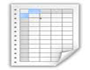Table 1

### Figures at a glanceFigure 1